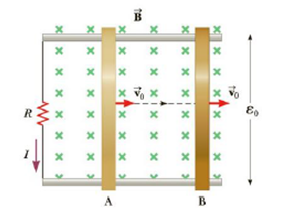Chapter 20, Problem 7CQ

Chapter
Section
Textbook Problem

Figure CQ20.7 shows a slidewire generator with motional cmf ε0 when the wire at A slides across the top and bottom rails at constant velocity v → 0. (a) When the wire reaches B so that the area enclosed by the circuit is doubled, determine the ratio of the new cmf to the original cmf, ε/ε0. (b) If the wire's speed is doubled so that v = 2v0 determine the ratio ε/ε0.Figure CQ20.7

(a)

To determine
To find the ratio of new emf to original emf if the area is doubled.

Explanation

If the area doubles lΔx becomes 2lΔx therefore the new emf becomes ε=2BlΔxΔt . Therefore the ratio of new emf to the original emf is given by

εε0

(b)

To determine
To find the ratio of new emf to original emf if the velocity is doubled.

Still sussing out bartleby?

Check out a sample textbook solution.

See a sample solution

The Solution to Your Study Problems

Bartleby provides explanations to thousands of textbook problems written by our experts, many with advanced degrees!

Get Started